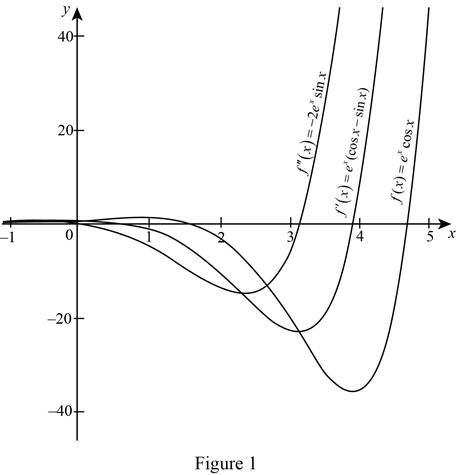# The derivative of f ( x ) and f ′ ( x ) .### Single Variable Calculus: Concepts...

4th Edition
James Stewart
Publisher: Cengage Learning
ISBN: 9781337687805### Single Variable Calculus: Concepts...

4th Edition
James Stewart
Publisher: Cengage Learning
ISBN: 9781337687805

#### Solutions

Chapter 3.3, Problem 26E

(a)

To determine

## To find: The derivative of f(x) and f′(x).

Expert Solution

The first derivative of f(x)=excosx is f(x)=ex(cosxsinx)_.

The second derivative of f(x)=excosx is f(x)=2exsinx_.

### Explanation of Solution

Given:

The function is f(x)=excosx.

Derivative rules:

(1) Product Rule: ddx[f(x)g(x)]=f(x)ddx[g(x)]+g(x)ddx[f(x)]

(2) Difference Rule: ddx[f(x)g(x)]=ddx[f(x)]ddx[g(x)]

Calculation:

Obtain the derivative f(x).

f(x)=ddx(f(x))=ddx(excosx)

Apply the product rule (1) and simplify further,

f(x)=exddx[cosx]+cosxddx[ex]=ex[sinx]+cosx[ex]=exsinx+excosx=ex(sinx+cosx)

Therefore, the first derivative of f(x)=excosx is f(x)=ex(cosxsinx)_.

Obtain the second derivative of f(x).

Apply the difference rule (2),

f(x)=ddx(f(x))=ddx(ex(sinx+cosx))=ddx((excosxexsinx))=(ddx(excosx)ddx(exsinx))

Apply the product rule (1),

f(x)=(exddx(cosx)+cosxddx(ex))(exddx(sinx)+sinxddx(ex))=(ex(sinx)+cosx(ex))(ex(cosx)+sinx(ex))=exsinx+excosxexcosxexsinx=2exsinx

Therefore, the derivative of f(x)=ex(sinx+cosx) is 2exsinx_.

(b)

To determine

### To check: The derivatives f(x), f′(x). and f″(x) are reasonable by comparing the graphs of f(x), f′(x). and f″(x).

Expert Solution

The derivatives are reasonable.

### Explanation of Solution

From part (a), the derivatives of f(x)=excosx are, f(x)=ex(cosxsinx) and f(x)=2exsinx.

Graph:

Use the online graphing calculator to draw the graph of the functions as shown below in Figure 1.Form Figure 1, it is observed that the function f(x) is continuous and monotonically decreasing on the positive real number.

The value of the derivative of the function at any point x can be estimated by drawing the tangent line at any point (x,f(x)) and then obtain the slope.

Mark the slope of the tangent as a point in y-axis and the value of x as a point in x-axis in the graph of f(x).

Proceed in the similar way at several points and obtain the rough graph of the f(x).

Hence, it can be concluded that the derivative of the function f(x) is reasonable.

The value of the derivative of the function at any point x can be estimated by drawing the tangent line at any point (x,f(x)) and then obtain the slope.

Mark the slope of the tangent as a point in y-axis and the value of x as a point in x-axis in the graph of f(x).

Proceed in the similar way at several points and obtain the rough graph of the f(x).

Hence, it can be concluded that the derivative of the function f(x) is reasonable.

### Have a homework question?

Subscribe to bartleby learn! Ask subject matter experts 30 homework questions each month. Plus, you’ll have access to millions of step-by-step textbook answers!